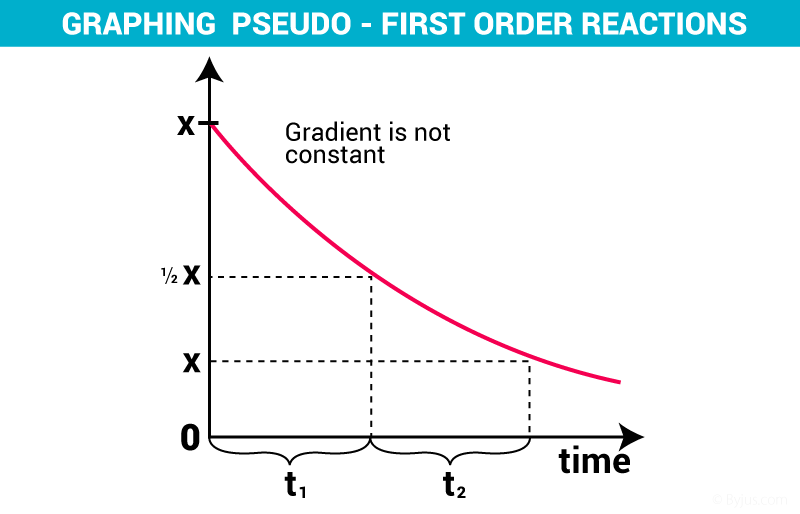Checkout JEE MAINS 2022 Question Paper Analysis : Checkout JEE MAINS 2022 Question Paper Analysis :

# Pseudo First Order Reaction

A Pseudo first-order reaction can be defined as a second-order or bimolecular reaction that is made to behave like a first-order reaction. This reaction occurs when one reacting material is present in great excess or is maintained at a constant concentration compared with the other substance.

In this section, we will learn about pseudo-first-order reaction and their rate equations. To begin with, reactions that appear to be second order in nature but are approximated as a first-order reaction on close analysis. For example, a second-order of the reaction is given by the equation,

A + B —-> C + D

This reaction is dependent upon the concentrations of both A and B but one of the components is present in large excess and thus its concentration hardly changes as the reaction proceeds.

So, if component B is in large excess and the concentration of B is very high as compared to that of A, the reaction is considered to be a pseudo-first-order reaction with respect to A and if component A is in large excess and the concentration of A is very high as compared to that of B, the reaction is considered to be pseudo-first order with respect to B.Pseudo First Order Reaction

## Example of First-Order Reaction

Here is an example to help you understand the concept more clearly.

Consider the hydrolysis of ethyl acetate, during the hydrolysis, the concentration of ethyl acetate is 0.02 mol/L whereas the amount of water is 20 mol/L as the process of hydrolysis involves a large amount of water. Let us say, the process of hydrolysis attains completion in time t.

The reaction can be represented as

CH3COOC2H5 + H2O —–> CH3COOH + C2H5OH

(ethyl acetate) (Water) (Acetic acid) (ethyl alcohol)

 Components CH3COOC2H5 H2O CH3COOH C2H5OH Concentration t=0 0.02 20 0 0 Concentration t 0 19.8 0.02 0.02

For the above-mentioned reaction, the rate equation can be given as,

Rate = k [CH3COOC2H5] [H2O]

Here we see that the concentration of water is very high and thus does not change much during the course of the reaction. Thus the rate of the reaction can be said to be independent of the change in the concentration of H2O. Putting the term for change of concentration of water in the above reaction to being zero we can write the effective rate equation as,

Rate = k [CH3COOC2H5]

Here, the term kt takes into account the value of the constant concentration of water.

where K = K’ [H2O]

We see that the reaction behaves as a first-order reaction. Such reactions are termed pseudo-first-order reactions.

Another example of pseudo-first-order reaction is the inversion of cane sugar, given by the following reaction,

C12H22O11 + H2O —–> C6H12O6 + C6H12O6

(Cane sugar) (Water) (Glucose) (Fructose)

The rate equation can be given as,

Rate = k [C12H22O11]

## Frequently Asked Questions – FAQs

### What is the definition of a first-order reaction?

A first-order reaction can be defined as a chemical reaction for which the reaction rate is entirely dependent on the concentration of only one reactant. In such reactions, if the concentration of the first-order reactant is doubled, then the reaction rate is also doubled. Similarly, if the first-order reactant concentration is increased five-fold, it will be accompanied by a 500% increase in the reaction rate.

### How we can conclude that the given reaction is pseudo first order reaction?

Pseudo first order reaction: The reaction which is bimolecular but order is one is called pseudo first order reaction. This happens when one of the reactants is in large excess. E.g., acidic hydrolysis of ester (ethyl acetate).
CH3COOC2H5 + H2O ——> CH3COOH + C2H5OH

### For a pseudo first-order reaction, what is the unit of the rate of the reaction?

The unit of the rate of the reaction (k) is (mol L-1)1-n s-1, where n is the order of the reaction.
For a pseudo first-order reaction n=1.
So, (mol L-1)1-n s-1 = (mol L-1)1-1 s-1 = s-1

### What are the examples of pseudo first order reaction?

Examples: 1. Acidic hydrolysis of ester (ethyl acetate).
CH3COOC2H5 + H2O ——> CH3COOH + C2H5OH
2. The inversion of cane sugar, given by the following reaction,
C12H22O11 + H2O —–> C6H12O6 + C6H12O6

### What is the graph of pseudo first order reaction?Test Your Knowledge On Pseudo First Order Reaction!

1. supun kahawatta

very good introduction about pseudo first order reaction.. I am really happy…

2. very good theory

3. devansh joshi

since when i m looking for byjus notes i had met with your deligent worker deepak on live chat and got appreciated very much by him
thanks to him and to byjus